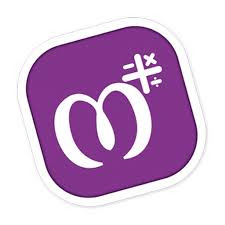# Download MAthry A way of Learning Math Supper Application from here

## Download MAthry A way of Learning Math Supper Application from here

Speed up mathematical calculations with Mathry!

A Simple yet amazing math application for students, teachers and parents.

Increase your brainpower using thousands of mathematical questions/quiz.Excellent for preschool and grade school kids to learn basic arithmetic ideas like Addition, Subtraction, Multiplication, Division, Decimals, Fractions, pure mathematics so on.Learn these basic operations:
✓ Addition
✓ Subtraction
✓ Multiplication
✓ Division

Other Features:
☆ Worksheet Generator (Teachers or Parents can use for an exam)
☆ Basic Operations on Number Base
☆ Fractions and Decimals
☆ Mixed Operators
☆ Percentage
☆ Square
☆ Square Root
☆ Cube
☆ Cube Root
☆ Find Missing
☆ and Much More!

Languages available:
» English
» Spanish
» Hindi
» Gujarati

This app is designed for Grade 1, Grade 2 and Grade 3 students to practice thousands of questions every day for free.

### IMPORTANT LINK::::

DOWNLOAD  MATHRY APPLICATION FROM HERE

We would like to cover all the math topics you can think about, this app will be constantly updated with fresh contents at the interval.

We are open to any suggestions and ideas, enjoy the Mathry.